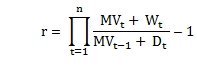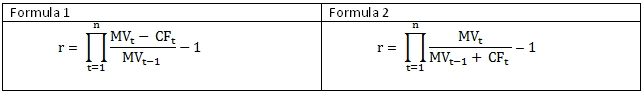## What’s new in XLeratorDB/financial ver 1.10

Dec 28

Written by: Charles Flock
12/28/2012 1:52 PMXLeratorDB / financial 1.10 contains 19 new functions: 3 functions for calculating time-weighted rate of return; 9 new functions for calculating some popular Capital Asset Pricing Model (CAPM) ratios; 3 new functions for calculating the interest rate sensitivity of a series of irregular cash flows; 2 new functions for calculating dates; a function for calculating multiple of invested capital; and a new function for calculating discounted cash flows.
We are happy to announce the availability of XLeratorDB / financial 2008 for SQL Server 2008 and SQL Server 2012 which contains the following new functions.
·         CFCONVEXITY – convexity for an irregular series of cash flows
·         CFDURATION – duration for an irregular series of cash flows
·         CFMDURATION – modified duration for an irregular series of cash flows
·         EQALPHA – alpha for a series of prices or valuations against a benchmark
·         EQBETA – beta for a series of prices or valuations against a benchmark
·         FIRSTWEEKDAY –first occurrence of a day of the week in a specified month
·         GTWRR – time-weighted rate of return using any one of three formulas
·         INFORATIO – Information ratio using return data
·         INFORATIO2 – Information ratio using price or valuation data
·         LASTWEEKDAY –last occurrence of a day of the week in a specified month
·         LMDIETZ – Modified Dietz for multiple period and links the results together
·         MOIC – multiple of invested capital
·         SHARPE – Sharpe ratio using return data
·         SHARPE2 – Sharpe ratio using price or valuation data
·         SORTINO – Sortino ratio using return data
·         TREYNOR – Treynor ratio using return data
·         TREYNOR2 –Treynor ratio using price or valuation data
·         TWROR – time-weighted rate of return with user-specified adjustments
·         XDCF – discounted cash flow as at a specific date
Time-weighted rate of return functions
XLeratorDB has had the TWRR function for over a year. The TWRR function uses the following formula to calculate returns.The new function, GTWRR, supports this formula and adds the following two new formulae.Where
r              time the time weighted rate of return
t              period for which the return is calculated
MV         ending market value for the period
D             amount that has been added to the portfolio during the period
W            amount that has been subtracted from the portfolio during the period
CF           net cash flow for the period
Additionally, GTWRR does not require a market value for every cash movement date and simply groups cash flows together between cash movement dates.
The LMDIETZ function is another way to calculate time-weighted rate of return. LMDIETZ calculates the Modified Dietz values for multiple periods and then computes the overall return for the multiple periods. This simplifies a process that we have previously written about.
There might be times when you need to specify which cash flows are combined with the ending market value for a period and which cash flows are combined with the beginning market value. The TWROR function allows you to do that.
Capital Asset Pricing Model
We have added 4 of the better-known ratios from the Capital Asset Pricing Model: Sharpe, Information, Treynor, and Sortino. In almost all of the literature that we could find, the ratios are calculated using return data and using the simple returns; in other words a simple arithmetic mean of the returns. The SHARPE, INFORATIO, TREYNOR, and SORTINO functions provide that calculation but also allow you to calculate using geometric returns rather than just simple returns.
We have also provided SHARPE2, INFORATIO2, and TREYNOR2 which calculate using price or valuation data rather than return data. These functions also support simple and geometric return calculations as well as providing the ability to calculate the ratio within and across portfolios.
Cash Flow Sensitivity Analysis
We have added the CFDURATION function to calculate the duration for a series of irregular cash flows. The CFDURATION is the equivalent of the DURATION function, which can only be used for bonds that pay regular, periodic interest. CFDURATION is the first derivative of the present value of the cash flows divided by the present value of the cash flows multiplied by 1 + discount rate.
The CFMDURATION function calculates the modified duration for a series of irregular cash flows. CFMDURATION is the equivalent of the MDURATION function, which can only be used for bonds that pay regular, periodic interest. CFMDURATION is CFDURATION divided by 1 + discount rate.
The CFCONVEXITY function calculates the convexity for a series of irregular cash flows. CFCONVEXITY is the equivalent of the CONVEXITY function which can only be used for bonds. CFCONVEXITY is calculated as the second derivative of the present value of the cash flows divided by the present value of the cash flows.
Discounted cash flows
XLeratorDB has had an XNPV function for over 4 years and it is modeled after the EXCEL XNPV function, except that the cash flows do not have to be in date order. We have finally decided that we don’t like the EXCEL implementation.
Let’s say you have a series of cash flows over the next 24 months and you want to discount them to the last day of this year (2012-12-31). To do this in EXCEL, you would have to include 2012-12-31 in your cash flows. And, since we modeled our XNPV function after EXCEL, you would have to do the same thing in SQL Server. And while that might be OK for EXCEL where you are dealing with a very small number of rows, what you really want to do is discount all the cash flows to a specified date, usually today.
This led to the creation of the XDCF function which separates out the valuation date from the cash flows which are being discounted. If you don’t feel like supplying the valuation date to the function, it will get the current date and use that.
Beta and Alpha
Many of our customers already us the SLOPE function to calculate the beta on the returns of individual stocks or on their portfolios (some customers might also use COVAR/VARP, but SLOPE is mathematically the same and more efficient). The SLOPE function makes this calculation very easy, provided that you have the return data, but it requires that the return data be calculated outside of the function and be stored somewhere so that it can be passed to the SLOPE function.
The EQBETA function allows you to pass the raw data into the function and it will automatically calculate the returns and return the beta value. Since we were doing beta, we also decided to add the EQALPHA function, which is comparable to the INTERCEPT function. Of course, EQBETA is slower than SLOPE since it has to calculate the returns as well as calculate the beta, but it’s faster than having to break out the calculation into two separate steps. If you have return data readily available, then you should continue to use the SLOPE function.
New date functions
In working with a client on a process to automatically bootstrap the zero-coupon yield curve on a SQL Server database, we realized that we had no easy to determine the start date or the end date for the futures contracts (which are the third Wednesday of the month). This lead to the development of the FIRSTWEEKDAY function which lets you calculate the first specified day of the week for any month. It accepts a numeric value for the day of the week, as well as the full name or abbreviated name of the day of the week, based upon the Windows Server regional settings. Note that this might not be the same as the language setting for the database.
We thought about having an additional parameter that would specify whether you wanted the first, second, third, fourth, or fifth occurrence of the day of the week within the month, but we felt that is was easier to use the DATEADD function in conjunction with the FIRSTWEEKDAY function since you know, for example, that the third Wednesday of a month is always 14 days after the first Wednesday. We did, however, create a LASTWEEKDAY function, so that you could calculate the last occurrence of a weekday within a specified month.
We hope that you find these functions very useful. You should read the documentation to find out more about specific functions that you might be interested in. You can try any and all of these functions out by downloading the 15-day free trial. If there is some function that you would like to add to the library, don’t be afraid to ask us about it.

All of these functions are available in XLeratorDB / finance 2008, XLeratorDB Suite 2008, XLeratorDB Developer 2008, and XLeratorDB SuitePLUS 2008 all of which run in SQL Server 2008 and SQL Server 2012.

Tags:
Categories:
Location: BlogsThe WestClinTech Blog

## Search Blogs

 KeywordsPhrase

## Blog Archives

Archive
 < December 2023 >
SunMonTueWedThuFriSat
262728293012
3456789
10111213141516
17181920212223
24252627282930
31123456
Monthly
Go

### SupportCopyright 2008-2023 Westclintech LLC         Privacy Policy        Terms of Service